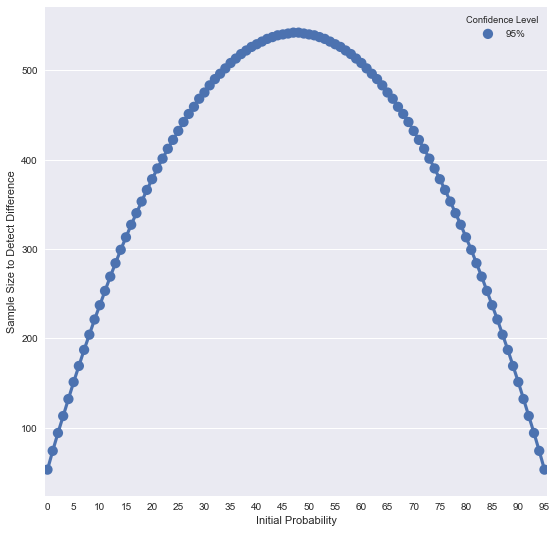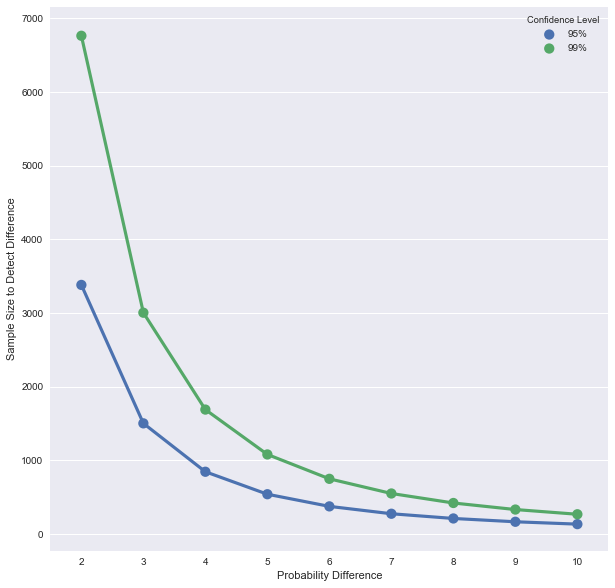# Using Python to Figure out Sample Sizes for your Study

It’s common wisdom among data scientists that 80% of your time is spent cleaning data, while 20% is the actual analysis.

There’s a similar issue when doing an empirical research study: typically, there’s tons of work to do up front before you get to the fun part (i.e. seeing and interpreting results).

One important up front activity in empirical research is figuring out the sample size you need. This is a crucial, since it significantly impacts the cost of your study and the reliability of your results. Collect too much sample: you’ve wasted money and time. Collect too little: your results may be useless.

Understanding the sample size you need depends on the statistical test you plan to use. If it’s a straightforward test, then finding the desired sample size can be just a matter of plugging numbers into an equation. However, it can be more involved, in which case a programming language like Python can make life easier. In this post, I’ll go through one of these more difficult cases.

Here’s the scenario: you are doing a study on a marketing effort that’s intended to increase the proportion of women entering your store (say, a change in signage). Suppose you want to know whether the change actually increased the proportion of women walking through. You’re planning on collecting the data before and after you change the signs and determine if there’s a difference. You’ll be using a two-proportion Z test for comparing the two proportions. You’re unsure how long you’ll need to collect the data to get reliable results – you first have to figure out how much sample you need!

### Overview of the Two Proportion Z test

The first step in determining the required sample size is understanding the statical test you’ll be using. The two sample Z test for proportions determines whether a population proportion p1 is equal to another population proportion p2. In our example, p1 and p2 are the proportion of women entering the store before and after the marketing change (respectively), and we want to see whether there was a statistically significant increase in p2 over p1, i.e. p2 > p1.

The test test the null hypothesis: p1 – p2 = 0. The test statistic we use to test this null hypotheses is:

$latex Z = \frac{p_2 – p_1}{\sqrt{p*(1-p*)(\frac{1}{n_1} + \frac{1}{n_2})}}&s=4$

Where p* is the proportion of “successes” (i.e. women entering the store) in the two samples combined. I.e.

$latex p* = \frac{n_1p_1 + n_2p_2}{n_1 + n_2}&s=4$

Z is approximately normally distributed (i.e. ~N(0, 1)), so given a Z score for two proportions, you can look up its value against the normal distribution to see the likelihood of that value occurring by chance.

So how to figure out the sample size we need? It depends on a few factors:

• The confidence level: How confident do we need to be to ensure the results didn’t occur by chance? For a given difference in results, detecting it with higher confidence requires more sample. Typical choices here include 95% or 99% confidence, although these are just conventions.
• The percentage difference that we want to be able to detect: The smaller the differences you want to be able to detect, the more sample will be required.
• The absolute values of the probabilities you want to detect differences on: This is a little trickier and somewhat unique to the particular test we’re working with. It turns out that, for example, detecting a difference between 50% and 51% requires a different sample size than detecting a difference between 80% and 81%. In other words, the sample size required is a function of p1, not just p1 – p2.
• The distribution of the data results: Say that you want to compare proportions within sub-groups (in our case, say you subdivide proportion of women by age group). This means that you need the sample to be big enough within each subgroup to get statistically significant comparisons. You often don’t know how the sample will pan out within each of these groups (it may be much harder to get sample for some). There are at least a couple of alternatives for you here: i) you could assume sample is distributed uniformly across subgroups ii) you can run a preliminary test (e.g. sit outside the store for half a day to get preliminary proportions of women entering for each age group).

So, how do you figure out sample sizes when there are so many factors at play?

### Figuring out Possibilities for Sample Sizes with Python

Ultimately, we want to make sure we’re able to calculate a difference between p1 and p2 when it exists. So, let’s assume you know that the “true” difference that exists between p1 and p2. Then, we can look at sample size requirements for various confidence levels and absolute levels of p1.

We need a way of figuring out Z, so we can determine whether a given sample size provides statistically significant results, so let’s define a function that returns the Z value given p1, p2, n1, and n2.

[gist https://gist.github.com/marknagelberg/b6bfe3e2141f8eeaeb0f2537ccbed81f /]

Then, we can define a function that returns the sample required, given p1 (the before probability), pdiff (i.e. p2 – p1), and alpha (which represents the p-value, or 1 minus the confidence level). For simplicity we’ll just assume that n1 = n2. If you know in advance that n1 will have about a quarter of the size of n2, then it’s trivial to incorporate this into the function. However, you typically don’t know this in advance and in our scenario an equal sample assumption seems reasonable.

The function is fairly simplistic: it counts up from n starting from 1, until n gets large enough where the probability of that statistic being that large (i.e. the p-value) is less than alpha (in this case, we would reject the null hypothesis that p1 = p2). The function uses the normal distribution available from the scipy library to calculate the p value and compare it to alpha.

[gist https://gist.github.com/marknagelberg/42b3077db573bcce55e00f975b8e4fc5 /]

These functions we’ve defined provide the main tools we need to determine minimum sample levels required.

As mentioned earlier, one complication to deal with is the fact that the sample required to determine differences between p1 and p2 depend on the absolute level of p1. So, the first question we want to answer is “what p1 that would require the biggest sample size to determine a given difference with p2?” Figuring this out allows you to calculate a lower bound on the sample you need for any p1. If you calculate the sample for the p1 with the highest required sample, you know it’ll be enough for any other p1.

Let’s say we want to be able to calculate a 5% difference with 95% confidence level, and we need to find a p1 that gives us the largest sample required. We first generate a list in Python of all the p1 to look at, from 0% to 95% and then use the sample_required function for each difference to calculate the sample.

[gist https://gist.github.com/marknagelberg/6b0679187cd7ce110546e581417bf6c4 /]

Then, we plot the data with the following code.

[gist https://gist.github.com/marknagelberg/2ace5e06f7012c76936887d82eff6aaf /]

Which produces this plot:This plot makes it clear that p1 = 50% produces the highest sample sizes.

Using this information, let’s say we want to calculate the sample sizes required to calculate differences in p1 and p2 where p2 – p1 is between  2% and 10%, and confidence levels are 95% or 99%. To ensure we get a sample large enough, we know to set p1 = 50%. We first write the code to build up the data frame to plot.

[gist https://gist.github.com/marknagelberg/4e647533f034a244ea3850e6ab5ec4da /]

Then we write the following code to plot the data with Seaborn.

[gist https://gist.github.com/marknagelberg/0c1845bf835da661851c948d837a0e95 /]

The final result is this plot:This shows the minimum sample required to detect probability differences between 2% and 10%, for both 95% and 99% confidence levels. So, for example, detecting a difference of 2% at 95% confidence level requires a sample of ~3,500, which translates into n1 = n2 = 1,750. So, in our example, you would need about 1,750 people walking into the store before the marketing intervention, and 1,750 people after to detect a 2% difference in probabilities at a 95% confidence level.

### Conclusion

The example shows how Python can be a very useful tool for performing “back of the envelope” calculations, such as estimates of required sample sizes for tests where this determination is not straightforward. These calculations can save you a lot of time and money, especially when you’re thinking about collecting your own data for a research project.

## 6 thoughts on “Using Python to Figure out Sample Sizes for your Study”

1.Elie Kawerk says:

Thanks for this nice post. I see that you did not include any power analysis here. I believe that the statsmodels library has some classes that can handle sample size calculation using power analysis without solely relying on confidence level calculations.

1.Mark Nagelberg says:

Thanks for the tip!!

2.Peter says:

Are you sure your sample sizes have sufficient power?

3.David Thompson says:

Would have been nice to have introduced PYTHON at the start, what is IT?, unless I missed it on my initial brief read. Will relook later when have more time

4.Chris says:

Thanks for this! Curious why you specify a range of differences and not just a specific % pdiff.

Cheers

5.Ksenia says:

Hi! Are you sure that n is the total number of people in both groups? The “sample_required” function returns n, which is n1=n2. So, isn’t it a number in one group?